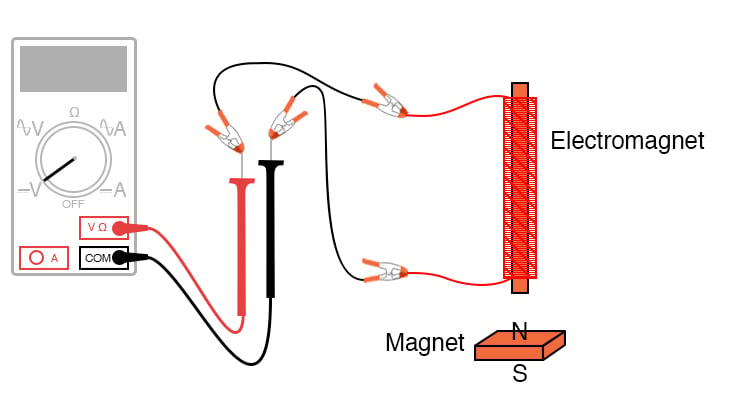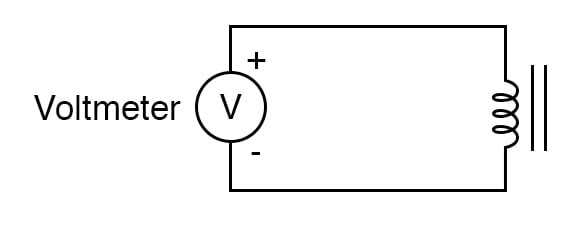Vol. DIY Electronics Projects
Chapter 2 Basic Projects and Test Equipment

# Intro Lab - Electromagnetic Induction

## In this hands-on electronics experiment, you will learn about electromagnetic induction using an electromagnet and a permanent magnet.

### Project Overview

Electromagnetic induction is a complementary phenomenon to electromagnetism. Instead of producing a magnetic field from electricity, we produce electricity from a magnetic field. There is one important difference, though, whereas electromagnetism produces a steady magnetic field from a steady electric current, electromagnetic induction requires motion between the magnet and the coil to produce a voltage. In this project, you measure electromagnetic induction using the test setup illustrated in Figure 1.### Learning Objectives

• Relationship between magnetic field strength and induced voltage

### Instructions

Step 1: Connect the multimeter to the coil, as illustrated in Figures 1 and 2, and set it to the most sensitive DC voltage range available.##### Figure 2. The schematic diagram for measuring the induced voltage from the electromagnet.

If you are using an analog multimeter, be sure to use long jumper wires and locate the meter far away from the coil, as the magnetic field from the permanent magnet may affect the meter’s operation and produce false readings. Digital meters are unaffected by magnetic fields.

Step 2: Measure the voltage output from the electromagnet. Hint: it should be zero!

Step 3: Move the magnet slowly to and from one end of the electromagnet, noting the polarity and magnitude of the induced voltage.

Step 4: Experiment with moving the magnet, and discover for yourself what factor(s) determine the amount of voltage induced. Consider the distance from the electromagnet and speed of movement.

Step 5: Repeat the process at the other end of the electromagnet coil and compare results.

Step 6: Repeat the process using the other end of the permanent magnet and compare.

### Related Content

Textbook:

Worksheets:

#### Lessons in Electric Circuits

Published under the terms and conditions of the Design Science License